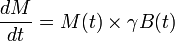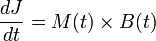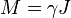# Bloch equations

(diff) ←Older revision | Current revision (diff) | Newer revision→ (diff)

Bloch equation describes evolution of classical magnetic moment in the magnetic field. The equation in the absence of T1 and T2 relaxation is:$\frac{dM}{dt} = M(t) \times \gamma B(t)$

## Summary explanation

A particle with a magnetic moment M in the magnetic field B will experience change of its mechanical angular moment J, i.e. torque = dJ/dt.$\frac{dJ}{dt} = M(t) \times B(t)$

Wigner-Eckart theorem in quantum mechanics proves that particle's magnetic moment is collinear with it's angular moment so that:$\displaystyle M = \gamma J$,

where γ is the scalar quantity called gyromagnetic ratio of the particle.

Therefore we have expression (known as Bloch Equation):$\frac{dM}{dt} = M(t) \times \gamma B(t)$